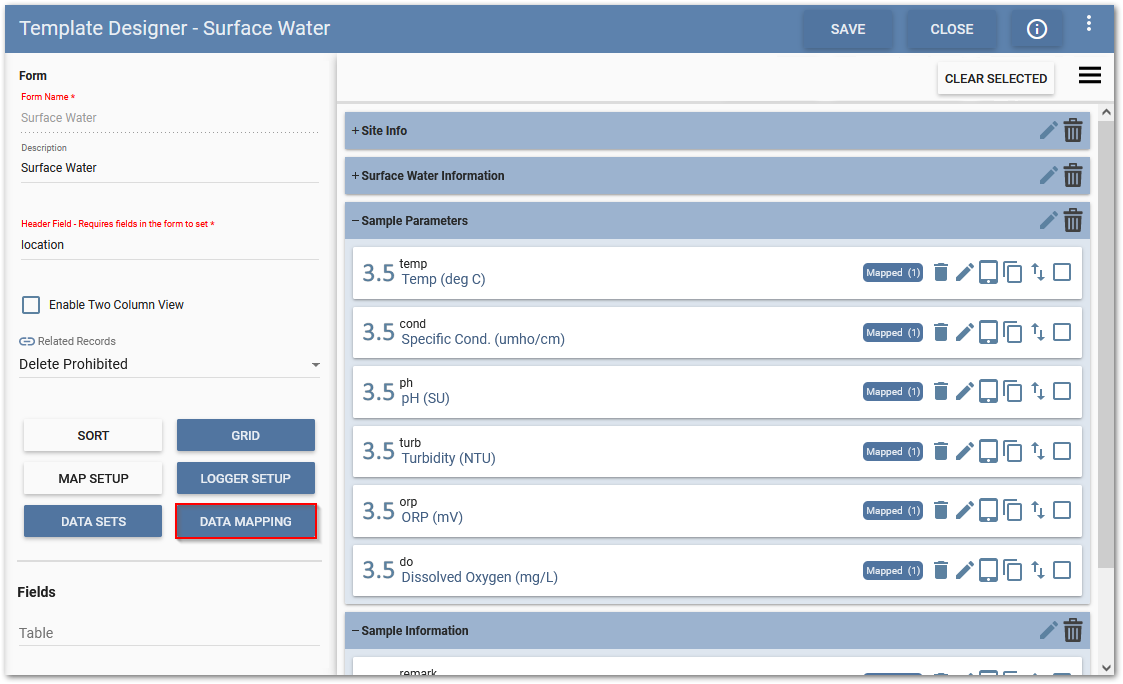﻿ Variables Attribute Example

# Variables Attribute Example

Variables is a data mapping attribute at the table level. The attribute is set to accept a JSON (JavaScript Object Notation) array, where the array values can be formatted using the following types: string, number, object, array, Boolean or null. The Variables feature iterates over each JSON object defined in the array, using the keys of the object as the field names to be replaced.

The variables attribute is useful when numerous data mappings with similar structures are required. Thus, this feature works well with parameter tables that contain a PARAM_CODE and PARAM_VALUE (e.g., Location_Parameter, SampleParameter_v1, etc.) to generate the data mappings and process the data without having to create multiple mapping tables for each field parameter (e.g. pH, ORP, DO, etc.) as described in this example article.

## Example

A Collect template has been assigned the EQEDD format and a form has been designed for surface water sampling.In the Data Mapping window for the SampleParameter_v1 section of the format, explicit values have been assigned for the SYS_SAMPLE_CODE and MEASUREMENT_DATE. The sample parameters, however, have not been assigned. Typically, field parameters (e.g., pH, Temp, ORP) would each have their own mapping table, but in this example the Variables feature is used instead.To assign the parameters to the Variables feature requires defining the mapping for PARAM_CODE and PARAM_VALUE. In this example, the PARAM_CODE value has been set to [X] and the PARAM_VALUE value has been set to [Y]. With the PARAM_CODE and PARAM_VALUE now defined, the JSON array can be set in the Variables feature.

 Note: The PARAM_CODE and PARAM_VALUE were arbitrarily chosen as [X] and [Y] and can be assigned any value but need to match the values in the Variables array string.For each record created, the Variables feature will replace the PARAM_CODE [X] with string values defined in the JSON objects. In this example, the string values "Temp", ''SpCond'', ''pH'', ''Turbidity'', ''ORP'', and "DO". The PARAM_VALUE will correspondingly replace the [Y] value with the associated field values entered in Collect Mobile. The Variables array can be extended to add as many objects as needed to match the number of parameters in the template.

Variables value in this example is: [{"x":"''Temp''","y":"[Temp]"},{"x":"''SpCond''","y":"[SC]"},{"x":"''pH''","y":"[pH]"},{"x":"''Turbidity''","y":"[Turb]"},{"x":"''ORP''","y":"[ORP]"},{"x":"''DO''","y":"[DO]"}]

 Note: The constant string values for the Variables are enclosed in Two Single Quotes (‘’) inside Double Quotes (“) as required for the JSON. Fields are in brackets ([ ]) inside Double Quotes (“).   The array formula for the Variables value needs to be created in a Word document or notepad file, then copied and pasted in the value section of the Variables attribute for the quotes to work correctly.